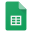# Motion

KinematicsReference - Forumla Sheet

## Activities & Topics

Labs & Activities

On Your Mark - Computer Graphing & Analysis of Toy Car's Motion

Gravity of the Situation - Finding the Value of Earth's Gravitational Acceleration

Newton's 2nd Law Apparatus Demo

Catch Me if You Can - Formulating and Discovering Your Meter-stick Grasp Response Time

Free Fall Apparatus Vacuum Tube - What factors affect the motion of objects?

Give It Some Gas - Send an Air-powered Rocket Upward to Calculate Its Motion

Topics Introduced

Vector and Scalar Quantities

Position

Displacement

Graphical Analysis - Slope & Area

Velocity

Acceleration

Free fall & Gravity

## Students are Expected to Understand

Measuring and calculating basic forms of linear motion

Creating and interpreting graphs on motion

Developing techniques on how to solve basic word problems

Observable patterns of free fall

### Some Notes on Motion

• There is a pattern to every moving object, and these patterns have been codified into equations to make these predictions easier when understood
• Any time an object moves, it will have a displacement and velocity. Displacement is how far it traveled directly from start to end while velocity includes the time it took
• Acceleration is how much of a difference there had been in velocities over a period of time. If the velocity stays the same, it means that no acceleration occurred
• Many of these measurements have a direction which are indicated by positive + and negative - signs. Moving forward, right, up is positive + while moving backward, left, or down is negative -
• Assume everything in this class is perfectly slippery. Ignore friction, drag, when resistance so we can focus on other ideas for now
• Everything we do in free fall is done on the Earth with a gravitational acceleration of about -10 m/s²
• Gravity makes everything accelerate any time anything is in the air

Common Challenges

• While an object may have moved, if it's displacement is zero, it effectively had no velocity
• Velocity is the not the same thing as speed, so get used to practicing using only velocity when speaking and recording
• When undergoing an acceleration, sometimes object stop moving for a moment, and even then, they still likely to be undergoing an acceleration

*These videos are to be used as a supplement and are not a replacement for in-class experiences# position-time graph

Is the slope positive or negative and what does the steepness of the graph tell you about how fast the object is moving?

What do you see during the first 5 seconds? The object moves +1 meter every second, making it +1 m/s

What do you see during the next 5 seconds? At time 5 s, the object is at the 5 m mark and stays at 5 m. If an object doesn't move, what is its velocity? 0m/s.

How are the last 2 to 3 seconds of the graph different than the first 5 seconds? What do you notice about the direction of the slope? Is this part of the graph more steep or less steep than before? We see that instead of pointing up, it points downward sharply, moving at -2 m every second, making it a negative velocity of about -2m/s.# velocity-time graph

A very different graph than a position vs. time graph. Each point on this graph no longer tells you were the object is but instead how fast and in which direction it is moving. Now, the slope shows acceleration

General Tips:

• A point along a v-t graph tells you the direction and velocity of the object at that time
• A slope of a v-t graph tells you the acceleration of the object during that time
• As the v-t graph moves further away from the x-axis, the faster the object is moving

Remember that velocity is no longer the slope but along the y-axis. Look at 0 s and try to see what velocity it has. The graph starts at 0 m/s meaning no velocity.

Is the object accelerating at 0 s? You can see that the velocity doesn't stay the same. It is definitely accelerating, and it is definitely possible to not be moving while accelerating!

There are two other points along this graph where there is no velocity while the object is still accelerating. Can you find these other two points? t = 30 s and 55 s

What the acceleration during the first 10 seconds? Take a look at the slope. The object is gaining positive velocity, and whenever the velocity changes, there is an acceleration. The velocity steadily climbs from 0 m/s to 60 m/s over 10 s, so it is means it's at 6 m/s²

Is the object not moving between 10 s and 15 s? At 10 s, the object is moving at 60 m/s and is moving, moving at the same rate of 60 m/s for the next five seconds. Because the velocity isn't changing, there is no acceleration

Think about this: is the velocity positive or negative at 20 s? Put your finger at the graph along the 20 s mark and slide your finger left until it hits the y-axis. What does it read? +40 m/s. At 20 s, the line is still in the positive part of the graph meaning that it has a positive velocity.

To follow up: is the slope positive or negative at 20 s and what does this slope mean? Remember that this slope is a slope of a velocity-time graph, that this velocity-time slope tells us acceleration, and this acceleration is negative

At 15 s to 30 s, the object never has a negative velocity. If you look carefully at the values, they are all positive until 30s when the object stops... and begins moving backwards, speeding up in the negative direction up to the 40s mark when it begins to slow. You can see the negative velocity continues for the rest of the graph until 55s.